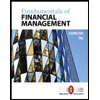Question

Currently working on finance practice problems and need help with setting up the problem, calculating it as well as understanding what points cause it to be either overvalued or undervalued

12) consider two securities, a and b. securities a and b have a correlation coefficient of 0.65. security a has a standard deviation of 12% and security b has standard deviation of 25% calculate the covariance between the two securities

a stock has a beta of the stock equalling to be 1.25. the risk free rate is 5% and the market risk premium is 6%. the estimated return for the stock is 14%. according to the CAPM you should

a) sell because it is overvalued

b) sell because it is undervalued

c) buy because it is overvalued

d) buy because it is undervalued

e) short because it is undervalued

Expert Solution

### Want to see the full answer?

Check out a sample Q&A hereStudents who’ve seen this question also like:Fundamentals of Financial Management, Concise Edition (MindTap Course List)
9th Edition
ISBN: 9781305635937
Author: Eugene F. Brigham, Joel F. Houston
Publisher: Cengage Learning